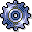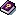(03) Cost-Based POC MethodUse

You can use the cost-based POC method for:

• Sales orders
• Projects

With the cost-based POC method you can:

• Calculate revenue affecting net income on the basis of the actual costs. This enables you to report a profit before any revenue has actually been received.
• Determine the revenue in excess of billings
• Show unrealized profits

The POC method is often used in Britain and North America. Because German law does not allow unrealized profits to be reported, the POC method is used in Germany only for internal information purposes.

Prerequisites

You have planned costs and revenues.

Choose a results analysis method in simplified Customizing for Product Cost by Sales Order under Period-End Closing ® Results Analysis ®Valuation Method.

Features

POC = C(a) / C(p)

C(PA) = POC * C(p) = C(a) / C(p) * C(p) = C(a)

R(PA) = POC * R(p) = C(a) / C(p) * R(p)

The calculated costs are equal to the actual costs. These costs can be passed to CO-PA together with the calculated revenue.

If the actual revenue is greater than the calculated revenue, the system creates a revenue surplus. The revenue surplus is basically a reserve.

If the actual revenue is less than the calculated revenue, the system creates revenue in excess of billings.

The calculated revenue and the revenue surplus can be transferred to FI and EC-PCA when you settle.

Example

You have planned a revenue of USD 200,000 and costs of USD 120,000 for a sales order.

Period 01

In period 01 you have actual costs of USD 20,000 but no revenues. In results analysis, the system calculates the following data:

• Costs affecting net income in the amount of the actual costs of USD 20,000
• Revenue affecting net income using the formula Calculated revenue = Actual costs / Planned costs x planned revenue of USD 33,333
• Revenue in excess of billings with the formula Revenue in excess of billings = Revenue affecting net income – Actual revenue

You then settle the following:

• The calculated revenue to CO-PA together with the actual costs
• The revenue in excess of billings to FI and EC-PCA

The following values are reported in CO-PA:

Profitability Analysis

 Calculated revenue 33,333 Cost of sales (actual costs) 20,000 Profit 13,333

The income statement shows the following values:

Income Statement

 Expense Revenue Actual costs 20,000 Actual revenue 0 Profit 13,333 Revenue in excess of billings 33,333 33,333 33,333

Although no revenues have been received, profit has already been capitalized.

Period 02

In period 02 the actual costs increase to USD 80,000. You deliver to your customer and send him a milestone invoice for USD 100,000. The order is partially delivered and partially billed. In results analysis, the system calculates the following data:

• Revenue affecting net income of USD 133,333
• Costs affecting net income in the amount of the actual costs of USD 80,000
• Revenue in excess of billings of USD 33,333

You then settle the following:

• The calculated revenue to CO-PA together with the actual costs
• The revenue in excess of billings to FI and EC-PCA

The following values are reported in CO-PA:

Profitability Analysis

 Calculated revenue 133,333 Cost of sales (actual costs) 80,000 Profit 53,333

The income statement shows the following values:

Income Statement

 Expense Revenue Actual costs 80,000 Actual revenue 100,000 Profit 53,000 Revenue in excess of billings 33,000 133,000 133,000

Profit is capitalized in the revenue in excess of billings. This results in a profit of USD 53,000.

Period 03

In period 03 the actual costs increase to USD 90,000. You deliver a second amount to your customer and send him a second milestone billing for USD 90,000. The total revenue is USD 190,000. The order is partially delivered and partially billed. In results analysis, the system calculates the following data:

• Costs affecting net income in the amount of the actual costs of USD 90,000
• Revenue affecting net income of USD 150,000
• A revenue surplus of USD 40,000 because the actual revenue exceeds the calculated revenue

You then settle the following:

• The calculated revenue to CO-PA together with the actual costs
• The revenue surplus to FI and EC-PCA

The following values are reported in CO-PA:

Profitability Analysis

 Calculated revenue 150,000 Cost of sales (actual costs) 90,000 Profit 60,000

The income statement shows the following values:

Income Statement

 Expense Revenue Actual costs 90,000 Actual revenue 190,000 Revenue surplus 40,000 Profit 60,000 190,000 190,000

Period 04

In period 04 the actual costs increase to USD 130,000. You deliver the remaining goods and send the customer the final invoice for USD 10,000. The total revenue is USD 200,000. The order is now fully delivered and fully invoiced.

In results analysis, the system calculates the following data:

• Costs affecting net income in the amount of the actual costs of USD 130,000
• Revenue affecting net income: USD 200,000 The calculated revenue is set equal to the actual revenue.

You then settle the following:

• The calculated revenue to CO-PA together with the actual costs
• The cancellation of the revenue surplus to FI and EC-PCA

The following values are reported in CO-PA:

Profitability Analysis

 Calculated revenue 200,000 Cost of sales (actual costs) 130,000 Profit 70,000

The income statement shows the following values:

Income Statement

 Expense Revenue Actual costs 130,000 Actual revenue 200,000 Profit 70,000 200,000 200,000

The order has a total profit of USD 70,000.